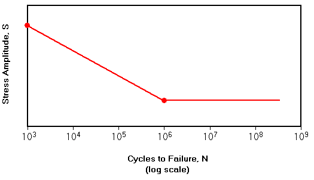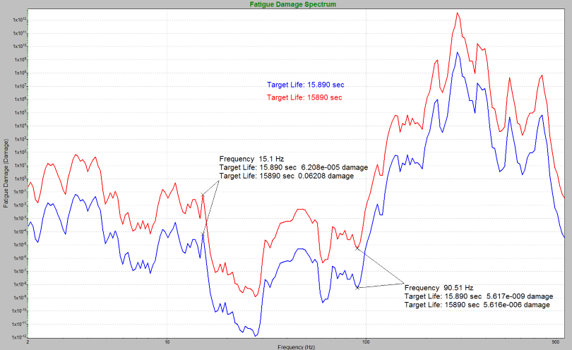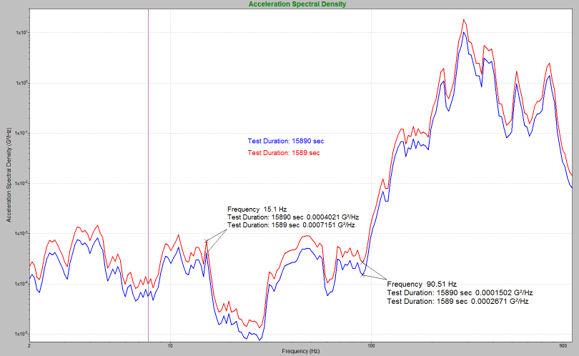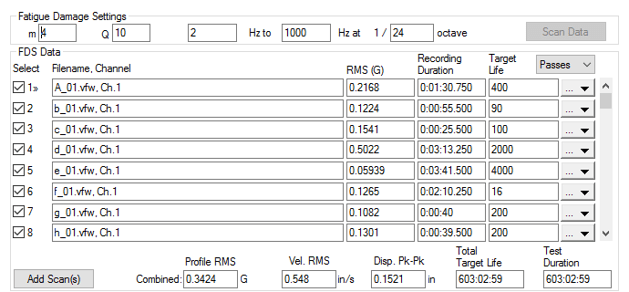# Acceleration Parameters for FDS

February 23, 2021

The fatigue damage spectrum (FDS) is used to create an accelerated random test that is the damage equivalent to the original environment. Before generating the FDS, the test engineer must set several acceleration parameters: the m value, resonance factor (Q), target life, and test duration.

### Acceleration Parameters and the S-N CurveThe S-N curve is a plot of stress (S) versus the number of cycles to failure (N) and is generally on a logarithmic scale. The S-N curve is based on the material, and a single S-N curve applies to the whole of the test. The slope of the S-N curve (b) is used to calculate the m value (m = -1/b).

From there, the calculation uses the m and Q values set by the engineer to determine the fatigue damage value at each given frequency. The combined damage values make up the FDS.

There are general values that can be used for m and Q, but more precise values can be determined.

#### The “m” Value

The m value is based on the slope of the S-N curve (b) and is, in most cases, some proportion of the negative reciprocal of the slope (-1/b). According to MIL-STD-810H, the m value is normally “80 percent of ‘-1/b’ for random waveshapes, and 60 percent of ‘-1/b’ for sinusoidal waveshapes” . A value between 5 and 8 is common.

A more specific m value can be found by generating an estimate of the S-N curve for a product. To do so, the engineer should test the product at different GRMS levels to failure and record the time required to reach failure. An approximation of the S-N curve can be back-calculated by plotting the data points on a log-log graph and then plotting the power-law model of the data. The slope of the power-law model is equal to b. The m value can be calculated using the negative reciprocal of the slope (-1/b).

FDS uses a stress-strain curve to determine test acceleration. Therefore, the accuracy of the m value determines the accuracy of the test acceleration. The importance of the accuracy of m increases as more acceleration is applied to the test.

Vibration Research created an m calculation tool that plots the GRMS vs. time to failure, determines the slope of the S-N curve, and calculates the m value based on the input data. The calculator can be downloaded here: FDS Calculator.

#### Consideration to Kurtosis

Real-world data are most often non-Gaussian. For non-Gaussian waveforms, a high m value gives more weight to high amplitude events than a lower m value. Thus, the high kurtosis peaks have more influence on the generation of the FDS. The result is a test with higher acceleration, velocity, and displacement than the original environment—i.e., a more conservative test.

An appropriate kurtosis level can be applied to a test to generate peak accelerations that are similar to the real world. The addition of kurtosis does not increase the relative damage of the test. When kurtosis is added, the overall GRMS is decreased to ensure that the relative damage remains equivalent to the original data sets.

#### Resonance Quality Factor (Q)

The resonance quality factor (Q) determines the damping value of an oscillation or the speed at which it decays. The Q value is equal to 0.5 divided by the critical damping ratio (ζ). A higher Q value will result in a lower rate of energy loss and slower decay of the oscillation. Conversely, a lower Q value will result in a higher rate of energy loss and faster decay of the oscillation.

The Q value for the FDS calculation should equal the Q value of the product’s sharpest resonance within the test frequency range. If the Q value of a product is not known, set the value to 10. A Q value of 10 can output an FDS-generated PSD with reasonable filtering and frequency response.

To calculate a more exact Q value for the product, run a simple sine sweep through the operating range of the device under test to determine the primary resonance. From there, the Q value of the resonance can be calculated.

The Q value has less effect on the FDS than the m value. A high Q value uses narrower filters, which results in sharper peaks. A low Q value results in a wider filter and a smoother spectrum but is less detailed.

#### Target Life and Test Duration

The target life determines the amount of time or number of cycles the time-history files should be run to represent one lifetime of damage. The value could be the length of a product warranty or the length of time the product should last when exposed to the imported time-history files.For multiple time-history files, the target life for the product is determined by weighing the individual time-history files by time or cycles. A target life value is assigned to each waveform to accomplish this.

When setting a test duration, the goal should not be to run the test in the quickest time possible. Less acceleration equates to a more accurate reflection of the true environment. In MIL-STD-810H, the following is advised:

“Provided that fatigue is a significant potential failure criterion for the materiel under test, this practice [test acceleration] is acceptable within strict limits, notably that test amplitudes are not over exaggerated (or accelerated) simply to achieve short test durations. Such excessive amplitudes may lead to wholly unrepresentative failures, and cause suppliers to design materiel to withstand arbitrary tests rather than the in-service conditions.”

Additionally, the instantaneous stress limit of the product should not be exceeded. MIL-STD-810H introduces the idea of an “exaggeration factor,” which should be “…kept to a minimum value consistent with the constraints of in-service time and desired time” .#### Octave Spacing

In the FDS, octave spacing defines the interval between frequency bins. Essentially, the value determines the number of breakpoints generated for the random PSD and the number of single/narrow frequency waveforms analyzed in the FDS calculation. For example, a 1/3 octave will result in fewer breakpoints and a wider band of content in each analysis bin compared to a 1/12 octave.

### Running the TestFatigue damage settings in VibrationVIEW.

The FDS-generated PSD is the damage equivalent to the lifetime of the product based on the imported files, m and Q values, target life, test duration, and kurtosis. The resulting PSD can be used in many stages of vibration testing, from initial product development to re-creating field failures in a lab environment.